## 前言#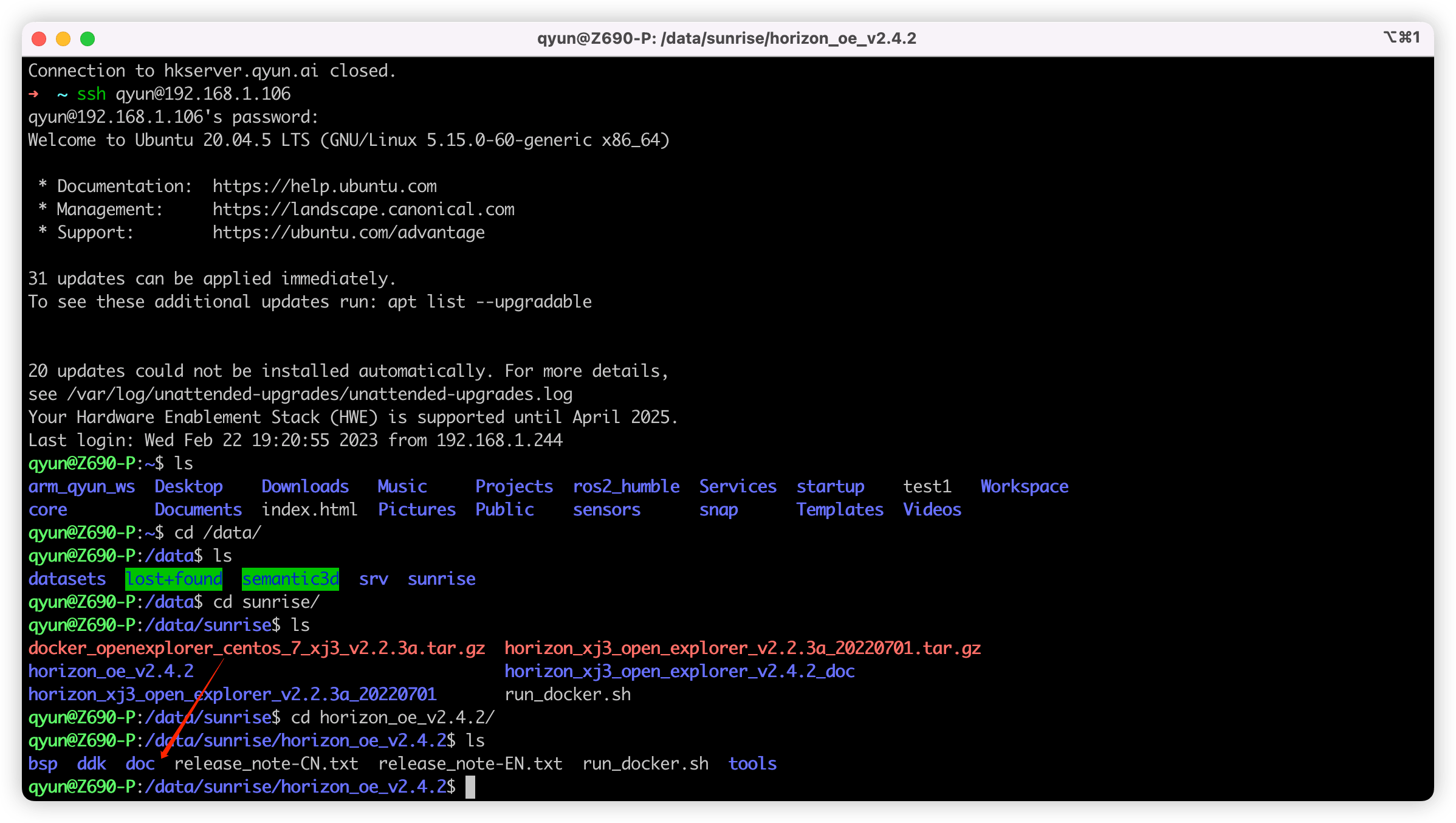``````python3 -m http.server 3000
``````

1. 训练的环境配置。

2. 如何基于官方的 COCO 数据集训练？这里就是指基于 mscoco 发布的包含 80 类的数据集

3. 如何基于自己的数据集进行训练？这里就是指自己建立的，自定义类别的，可能只有四五类，没有 80 类的数据集。

## 训练的环境配置#

OS: Ubuntu 20.04

Docker: 20.10.23

Nvidia Docker: 2.11.0-1

GPU: RTX3090

NVIDIA Driver Version: 515.65.01

CUDA Version: 11.7

OE Version: v2.4.2( gcc-9.3.0 For XJ3 )

``````#!/bin/bash
export version=v2.4.2

export ai_toolchain_package_path=/data/sunrise/horizon_oe_v2.4.2

export dataset_path=/data/datasets

docker run -dt --runtime=nvidia -e NVIDIA_DRIVER_CAPABILITIES=compute,utility \
-e NVIDIA_VISIBLE_DEVICES=all --shm-size="15g" \
--restart=always --network=host \
-v "\$ai_toolchain_package_path":/open_explorer \
-v "\$dataset_path":/data \
openexplorer/ai_toolchain_centos_7_xj3:"\${version}"
``````

`version` 代表 Docker 镜像版本，可选版本参考：资料下载专区

`ai_toolchain_package_path` 代表 OE 开发包的路径，指定 OE 包中 ai_toolchain 的路径也可以，但是我建议把整个 OE 包都挂进去得了，没啥差别。

`dataset_path` 代表训练需要的数据集路径，这里可以新建一个空的文件夹然后挂载进去，也可以把你所有的数据集都准备好，然后放到一个文件夹，然后挂进去，需要训练哪个后面指定哪个即可，我们这里方便起见可以选择前者（官方说如果这里指定为空可能会出问题，但是我测试发现挂载空文件夹不会有问题）。

`docker run` 部分就是启动一个 Docker 容器，其中比较重要的参数： `-dt` 可以让容器使用 Daemon 的方式启动一个交互式终端，可以保证容器一直存活不会 detach 后就被杀死，方便我们多次操作；`--restart=always` 可以在每次开机时自动重启容器，可以视自己的情况去掉或者改成 `--restart=unless-stopped` 等；`--network=host` 指定与宿主机器使用同一个网络，方便我们查看文档或者后面的网络结构。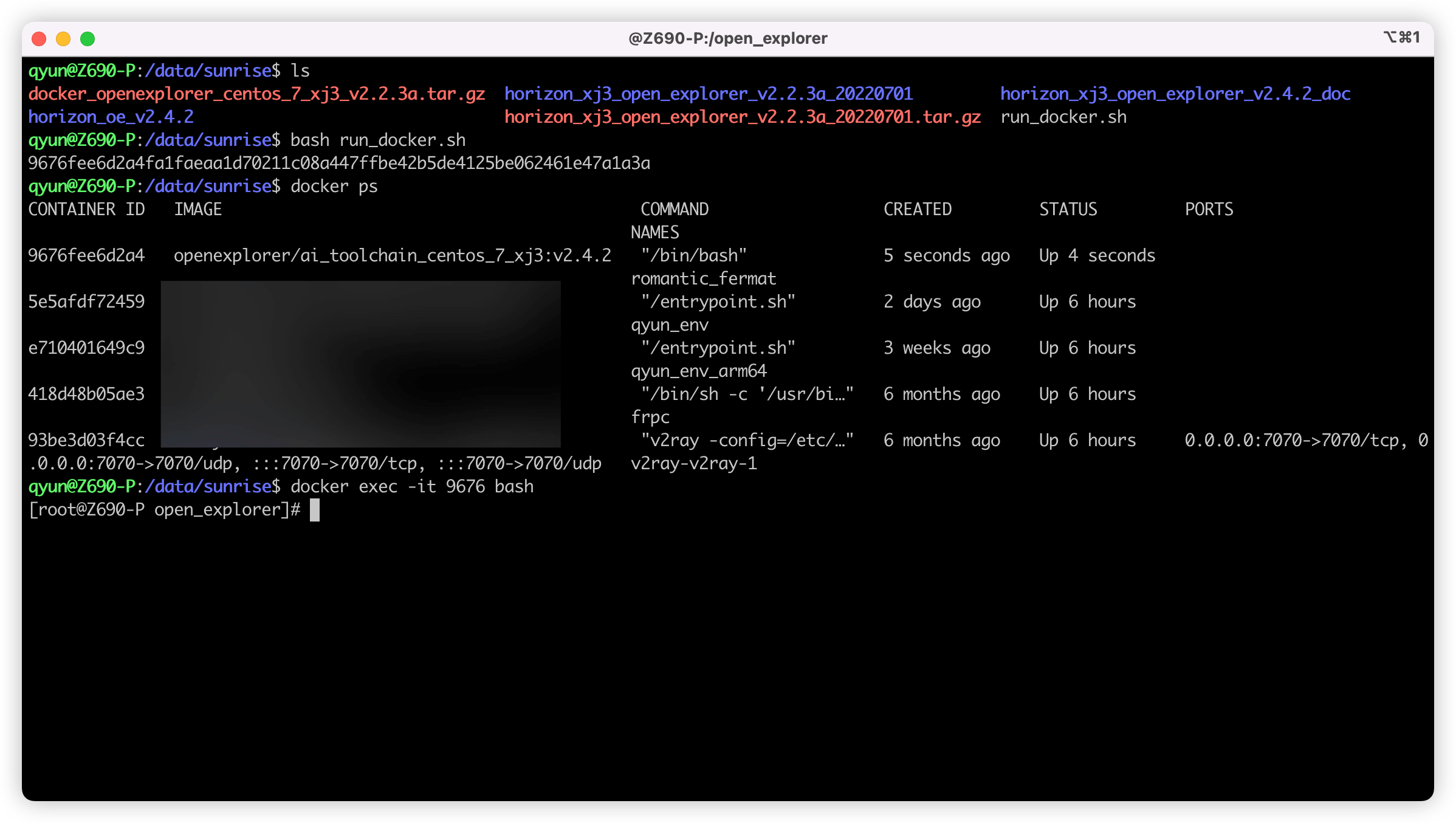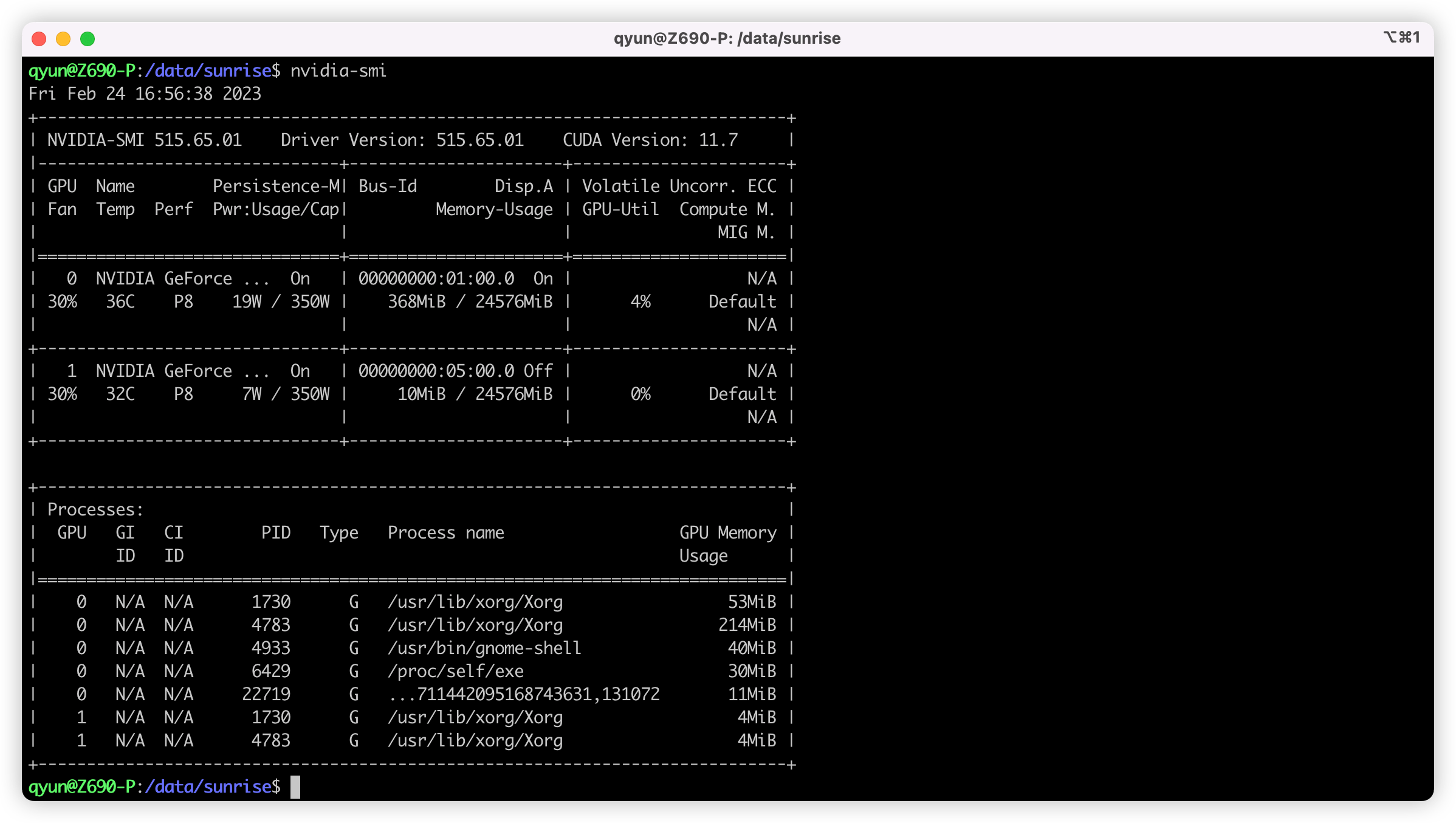## 训练 COCO 数据集并进行部署#

### 数据准备#

``````# 进入之前挂载的 /data 目录
cd /data
# 创建一个目录来存储数据集
mkdir mscoco
# 下载 train2017.zip，即训练数据集
wget -c http://images.cocodataset.org/zips/train2017.zip
# 下载 val2017.zip，即验证数据集
wget -c http://images.cocodataset.org/zips/val2017.zip
# 下载 annotations_trainval2017.zip 即对应标签
wget -c http://images.cocodataset.org/annotations/annotations_trainval2017.zip
# 解压
unzip train2017.zip
unzip val2017.zip
unzip annotations_trainval2017.zip
``````

``````# 转换训练集
python3 tools/datasets/mscoco_packer.py --src-data-dir /data/mscoco/ --target-data-dir /data/mscoco --split-name train --pack-type lmdb
# 转换验证集
python3 tools/datasets/mscoco_packer.py --src-data-dir /data/mscoco/ --target-data-dir /data/mscoco --split-name val --pack-type lmdb
``````

### 修改配置文件#

``````cp fcos_efficientnetb0_mscoco.py fcos_efficientnetb0_mscoco_back.py
``````

1. `device_ids`：这里代表的是你使用的 GPU 序号，如果只有一个 GPU 就只保留 ，两个就是 [0, 1]，以此类推。
2. 所有跟 `./tmp_data` 有关的位置，这里相关的都是指定的数据集位置，官方举例是将数据集都放在了同 configs 同目录的 `tmp_data` 目录，但是我们放在了 `/data` 目录，因此要将所有的 `./tmp_data` 都改成 `/data`
3. `float_trainer`：这个参数是个 Dict，里面包含了一个 `num_epochs `，代表我们训练多少轮，这里默认是 300，可以根据自己需求调整，例如 5、10、100 等。

### 开始训练！#

``````# 进入 horizon_model_train_samples 目录
cd /open_explorer/ddk/samples/ai_toolchain/horizon_model_train_samples
# 开始训练
python3 tools/train.py --step float --config configs/detection/fcos/fcos_efficientnetb0_mscoco.py
``````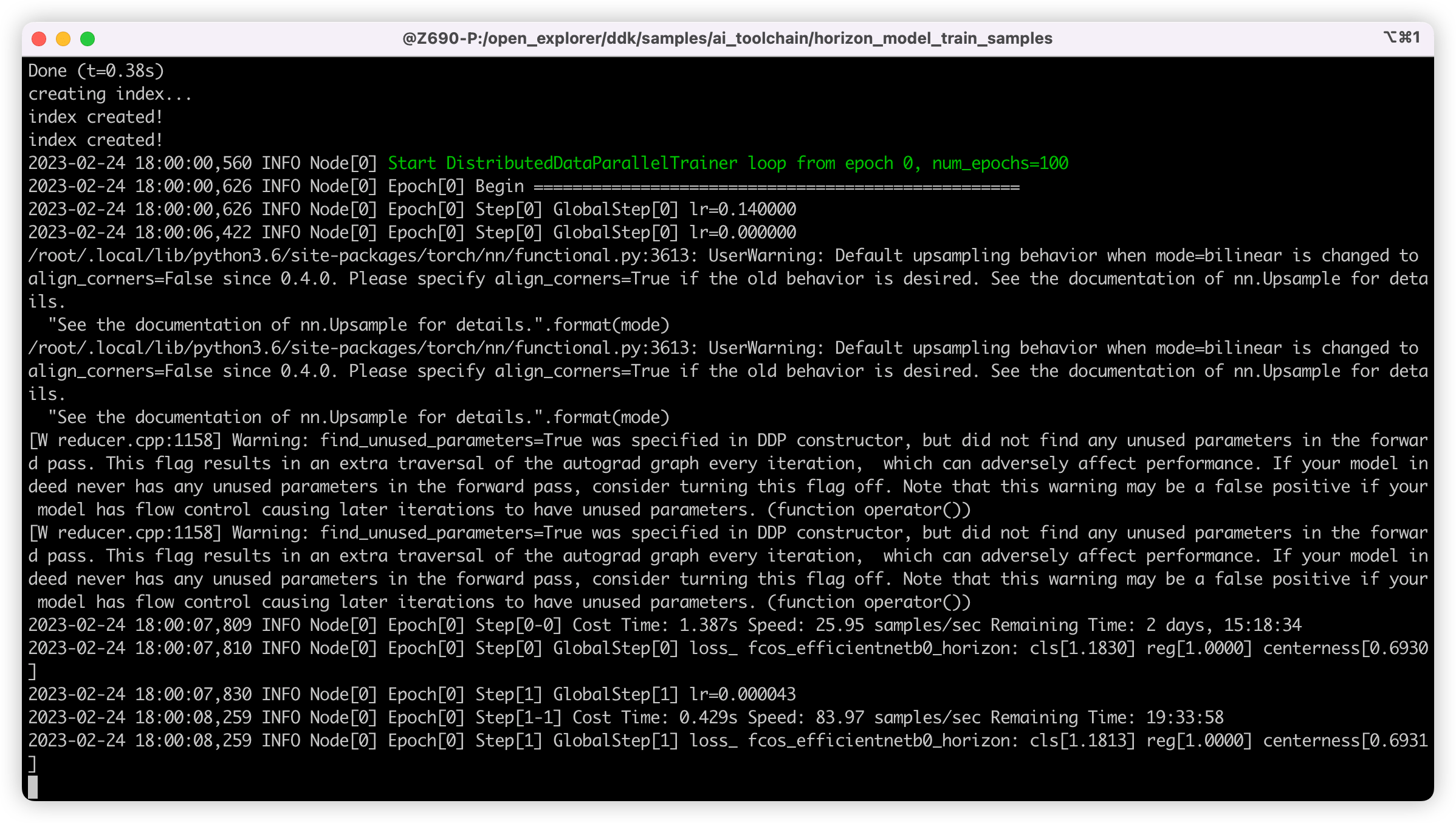### 模型转换#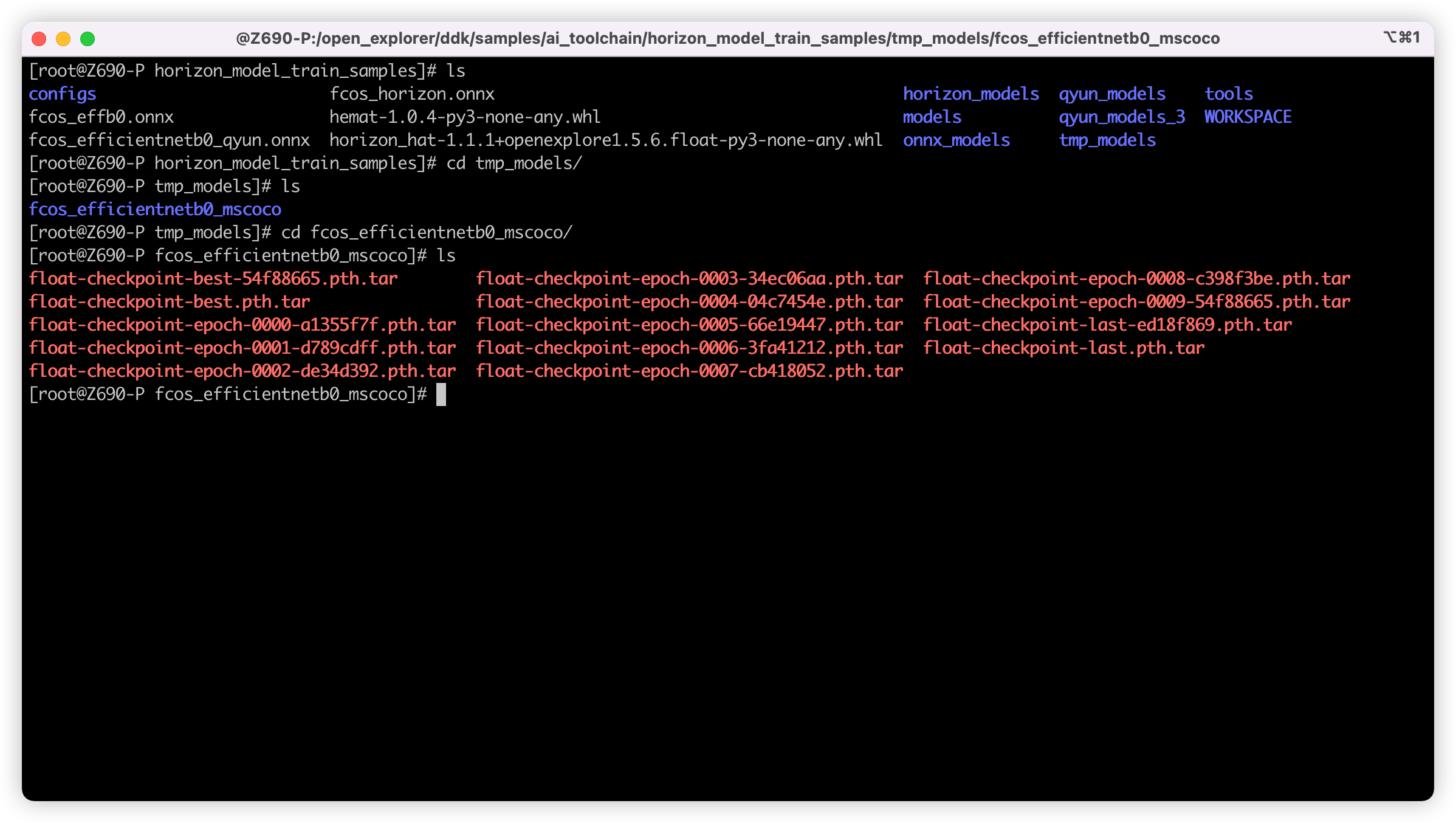``````python3 tools/export_onnx.py --config configs/detection/fcos/fcos_efficientnetb0_mscoco.py --ckpt tmp_models/fcos_efficientnetb0_mscoco/float-checkpoint-best.pth.tar --onnx-name fcos.onnx
``````

``````# 进入 horizon_model_train_samples 目录
cd /open_explorer/ddk/samples/ai_toolchain/horizon_model_train_samples
# 建立文件夹
mkdir -p ../horizon_model_convert_sample/08_customs
# 移动 onnx 模型过去
cp ./fcos.onnx ../horizon_model_convert_sample/08_customs
``````

• 01_check.sh
``````# 01_check.sh
set -e -v
cd \$(dirname \$0)

model_type="onnx"
onnx_model="../../../08_customs/fcos.onnx"
march="bernoulli2"

hb_mapper checker --model-type \${model_type} \
--model \${onnx_model} \
--march \${march}
``````
• 02_preprocess.sh
``````# 02_preprocess.sh
set -e -v

cd \$(dirname \$0) || exit

python3 ../../../data_preprocess.py \
--src_dir /data/mscoco/train2017 \
--dst_dir ./calibration_data_yuv_f32 \
--cal_img_num 50 \
--pic_ext .yuv \
--saved_data_type float32
``````
• fcos_efficientnetb0_config.yaml
``````# fcos_efficientnetb0_config.yaml
model_parameters:
onnx_model: '../../../08_customs/fcos.onnx'
march: "bernoulli2"
layer_out_dump: False
working_dir: 'fcos_test'
output_model_file_prefix: 'fcos_test'
input_parameters:
input_name: ""
input_type_rt: 'nv12'
input_type_train: 'yuv444'
input_layout_train: 'NCHW'
input_shape: ''
norm_type: 'data_mean_and_scale'
mean_value: 128.0
scale_value: 0.0078125
calibration_parameters:
cal_data_dir: './calibration_data_yuv_f32_qyun'
cal_data_type: 'float32'
calibration_type: 'max'
max_percentile: 0.99996
compiler_parameters:
compile_mode: 'latency'
debug: False
optimize_level: 'O3'
``````

### 模型推理#

``````#!/usr/bin/env python3

import os
from hobot_dnn import pyeasy_dnn as dnn
from hobot_vio import libsrcampy as srcampy
import numpy as np
import cv2
import colorsys
from time import time

# detection model class names

def get_classes():
return np.array(["person", "bicycle", "car",
"motorcycle", "airplane", "bus",
"train", "truck", "boat",
"traffic light", "fire hydrant", "stop sign",
"parking meter", "bench", "bird",
"cat", "dog", "horse",
"sheep", "cow", "elephant",
"bear", "zebra", "giraffe",
"backpack", "umbrella", "handbag",
"tie", "suitcase", "frisbee",
"skis", "snowboard", "sports ball",
"kite", "baseball bat", "baseball glove",
"skateboard", "surfboard", "tennis racket",
"bottle", "wine glass", "cup",
"fork", "knife", "spoon",
"bowl", "banana", "apple",
"sandwich", "orange", "broccoli",
"carrot", "hot dog", "pizza",
"donut", "cake", "chair",
"couch", "potted plant", "bed",
"dining table", "toilet", "tv",
"laptop", "mouse", "remote",
"keyboard", "cell phone", "microwave",
"oven", "toaster", "sink",
"refrigerator", "book", "clock",
"vase", "scissors", "teddy bear",
"hair drier", "toothbrush"])
# return np.array(["tissue", "sock", "shoe", "cable", "bin"])

# bgr格式图片转换成 NV12格式

def bgr2nv12_opencv(image):
height, width = image.shape, image.shape
area = height * width
yuv420p = cv2.cvtColor(
image, cv2.COLOR_BGR2YUV_I420).reshape((area * 3 // 2,))
y = yuv420p[:area]
uv_planar = yuv420p[area:].reshape((2, area // 4))
uv_packed = uv_planar.transpose((1, 0)).reshape((area // 2,))

nv12 = np.zeros_like(yuv420p)
nv12[:height * width] = y
nv12[height * width:] = uv_packed
return nv12

def postprocess(model_output,
model_hw_shape,
origin_image=None,
origin_img_shape=None,
score_threshold=0.5,
nms_threshold=0.6,
dump_image=False):
input_height = model_hw_shape
input_width = model_hw_shape
if origin_image is not None:
origin_image_shape = origin_image.shape[0:2]
else:
origin_image_shape = origin_img_shape

prediction_bbox = decode(outputs=model_output,
score_threshold=score_threshold,
origin_shape=origin_image_shape,
input_size=512)

prediction_bbox = nms(prediction_bbox, iou_threshold=nms_threshold)

prediction_bbox = np.array(prediction_bbox)
topk = min(prediction_bbox.shape, 1000)

if topk != 0:
idx = np.argpartition(prediction_bbox[..., 4], -topk)[-topk:]
prediction_bbox = prediction_bbox[idx]

if dump_image and origin_image is not None:
draw_bboxs(origin_image, prediction_bbox)
return prediction_bbox

def draw_bboxs(image, bboxes, gt_classes_index=None, classes=get_classes()):
"""draw the bboxes in the original image
"""
num_classes = len(classes)
image_h, image_w, channel = image.shape
hsv_tuples = [(1.0 * x / num_classes, 1., 1.) for x in range(num_classes)]
colors = list(map(lambda x: colorsys.hsv_to_rgb(*x), hsv_tuples))
colors = list(
map(lambda x: (int(x * 255), int(x * 255), int(x * 255)),
colors))

fontScale = 0.5
bbox_thick = int(0.6 * (image_h + image_w) / 600)

for i, bbox in enumerate(bboxes):
coor = np.array(bbox[:4], dtype=np.int32)

if gt_classes_index == None:
class_index = int(bbox)
score = bbox
else:
class_index = gt_classes_index[i]
score = 1

bbox_color = colors[class_index]
c1, c2 = (coor, coor), (coor, coor)
cv2.rectangle(image, c1, c2, bbox_color, bbox_thick)
classes_name = classes[class_index]
bbox_mess = '%s: %.2f' % (classes_name, score)
t_size = cv2.getTextSize(bbox_mess,
0,
fontScale,
thickness=bbox_thick // 2)
cv2.rectangle(image, c1, (c1 + t_size, c1 - t_size - 3),
bbox_color, -1)
cv2.putText(image,
bbox_mess, (c1, c1 - 2),
cv2.FONT_HERSHEY_SIMPLEX,
fontScale, (0, 0, 0),
bbox_thick // 2,
lineType=cv2.LINE_AA)
print("{} is in the picture with confidence:{:.4f}".format(
classes_name, score))
#    cv2.imwrite("demo.jpg", image)
return image

def decode(outputs, score_threshold, origin_shape, input_size=512):
def _distance2bbox(points, distance):
x1 = points[..., 0] - distance[..., 0]
y1 = points[..., 1] - distance[..., 1]
x2 = points[..., 0] + distance[..., 2]
y2 = points[..., 1] + distance[..., 3]
return np.stack([x1, y1, x2, y2], -1)

def _scores(cls, ce):
cls = 1 / (1 + np.exp(-cls))
ce = 1 / (1 + np.exp(-ce))
return np.sqrt(ce * cls)

def _bbox(bbox, stride, origin_shape, input_size):
# l t r b | h, w = t, r
h, w = bbox.shape[1:3]
yv, xv = np.meshgrid(np.arange(h), np.arange(w))
xy = (np.stack((yv, xv), 2) + 0.5) * stride
bbox = _distance2bbox(xy, bbox)
# opencv read, shape is w, shape is h
scale_w = origin_shape / input_size
scale_h = origin_shape / input_size
scale = max(origin_shape, origin_shape) / input_size
# origin img is pad resized
# bbox = bbox * scale
# origin img is resized
bbox = bbox * [scale_w, scale_h, scale_w, scale_h]
return bbox

bboxes = list()
strides = [8, 16, 32, 64, 128]

# 各个 stride 找符合的模型
for i in range(len(strides)):
cls = outputs[i].buffer
bbox = outputs[i + 5].buffer
ce = outputs[i + 10].buffer
scores = _scores(cls, ce)

classes = np.argmax(scores, axis=-1)
classes = np.reshape(classes, [-1, 1])
max_score = np.max(scores, axis=-1)
max_score = np.reshape(max_score, [-1, 1])
bbox = _bbox(bbox, strides[i], origin_shape, input_size)
bbox = np.reshape(bbox, [-1, 4])

pred_bbox = np.concatenate([bbox, max_score, classes], axis=1)

index = pred_bbox[..., 4] > score_threshold
pred_bbox = pred_bbox[index]
bboxes.append(pred_bbox)

return np.concatenate(bboxes)

def nms(bboxes, iou_threshold, sigma=0.3, method='nms'):
def bboxes_iou(boxes1, boxes2):
boxes1 = np.array(boxes1)
boxes2 = np.array(boxes2)
boxes1_area = (boxes1[..., 2] - boxes1[..., 0]) * \
(boxes1[..., 3] - boxes1[..., 1])
boxes2_area = (boxes2[..., 2] - boxes2[..., 0]) * \
(boxes2[..., 3] - boxes2[..., 1])
left_up = np.maximum(boxes1[..., :2], boxes2[..., :2])
right_down = np.minimum(boxes1[..., 2:], boxes2[..., 2:])
inter_section = np.maximum(right_down - left_up, 0.0)
inter_area = inter_section[..., 0] * inter_section[..., 1]
union_area = boxes1_area + boxes2_area - inter_area
ious = np.maximum(1.0 * inter_area / union_area,
np.finfo(np.float32).eps)

return ious

classes_in_img = list(set(bboxes[:, 5]))
best_bboxes = []

for cls in classes_in_img:
cls_mask = (bboxes[:, 5] == cls)

while len(cls_bboxes) > 0:
max_ind = np.argmax(cls_bboxes[:, 4])
best_bbox = cls_bboxes[max_ind]
best_bboxes.append(best_bbox)
cls_bboxes = np.concatenate(
[cls_bboxes[:max_ind], cls_bboxes[max_ind + 1:]])
iou = bboxes_iou(best_bbox[np.newaxis, :4], cls_bboxes[:, :4])
weight = np.ones((len(iou),), dtype=np.float32)

assert method in ['nms', 'soft-nms']

if method == 'nms':
if method == 'soft-nms':
weight = np.exp(-(1.0 * iou ** 2 / sigma))

cls_bboxes[:, 4] = cls_bboxes[:, 4] * weight
score_mask = cls_bboxes[:, 4] > 0.

return best_bboxes

def print_properties(pro):
print("tensor type:", pro.tensor_type)
print("data type:", pro.dtype)
print("layout:", pro.layout)
print("shape:", pro.shape)

if __name__ == '__main__':
# 打印输入 tensor 的属性
print_properties(models.inputs.properties)
# 打印输出 tensor 的属性
print(len(models.outputs))
for output in models.outputs:
print_properties(output.properties)

if frame is None:
print("Image is not exist.")
height, width = frame.shape[:2]
print("height: ", height, "width: ", width)
# 把图片缩放到模型的输入尺寸
# 获取算法模型的输入tensor 的尺寸
h, w = models.inputs.properties.shape, models.inputs.properties.shape
des_dim = (w, h)
resized_data = cv2.resize(frame, des_dim, interpolation=cv2.INTER_AREA)
nv12_data = bgr2nv12_opencv(resized_data)

# Forward
outputs = models.forward(nv12_data)
# Do post process
input_shape = (h, w)
prediction_bbox = postprocess(
outputs, input_shape, origin_img_shape=(height, width))

if frame.shape != height or frame.shape != width:
frame = cv2.resize(frame, (width, height),
interpolation=cv2.INTER_AREA)

# Draw bboxs
box_bgr = draw_bboxs(frame, prediction_bbox)

cv2.imwrite("kite_with_bbox.jpg", box_bgr)
``````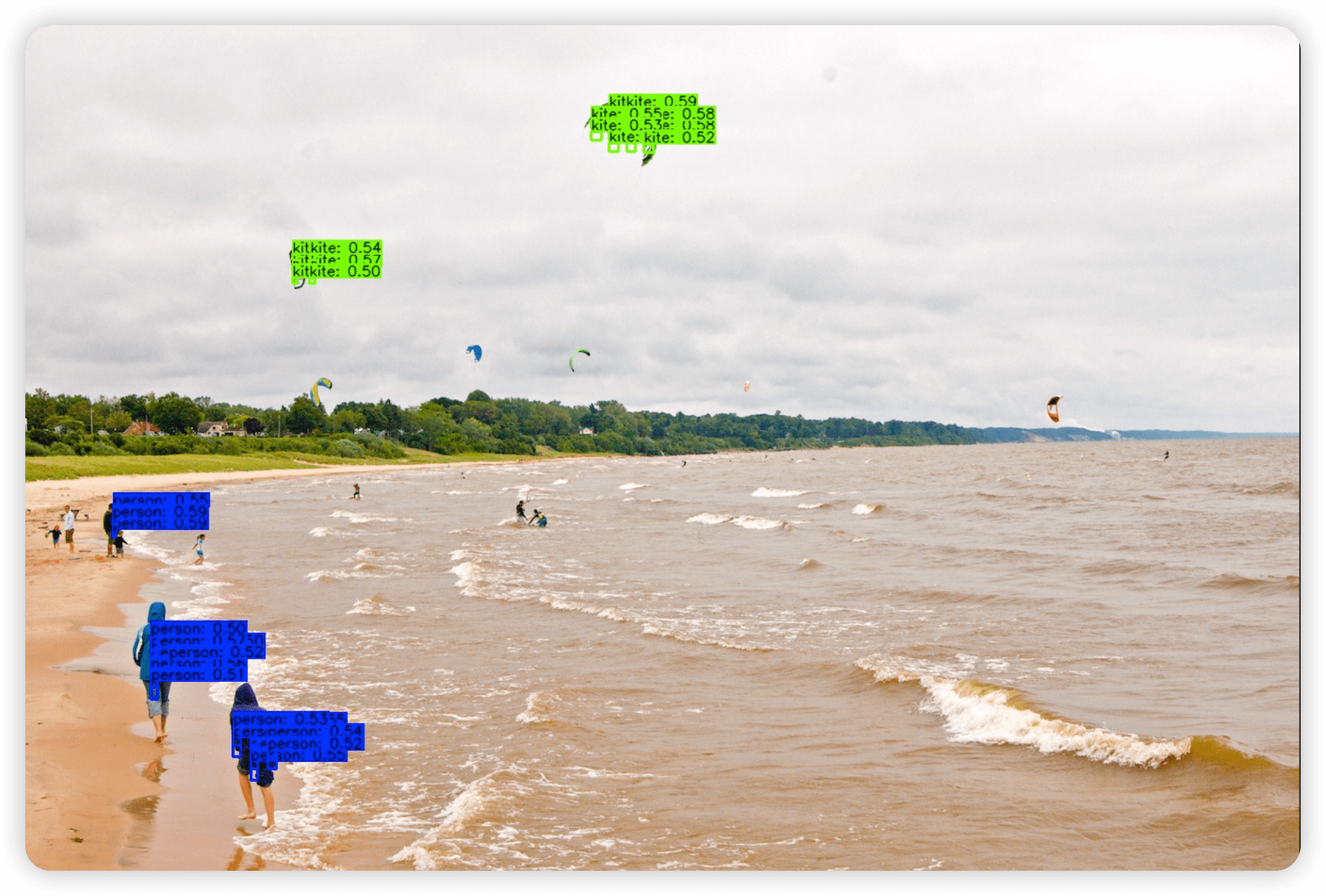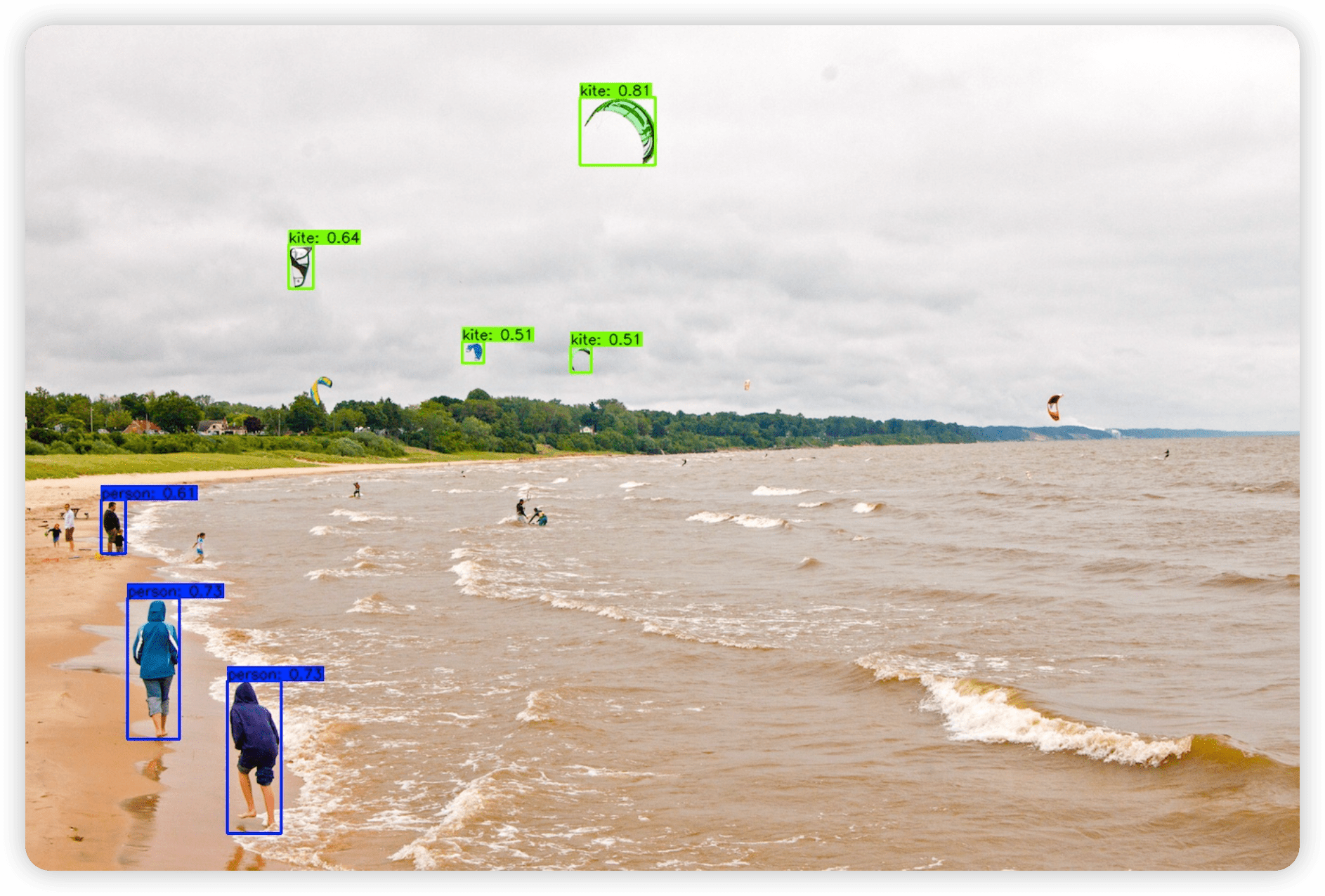### 查找问题#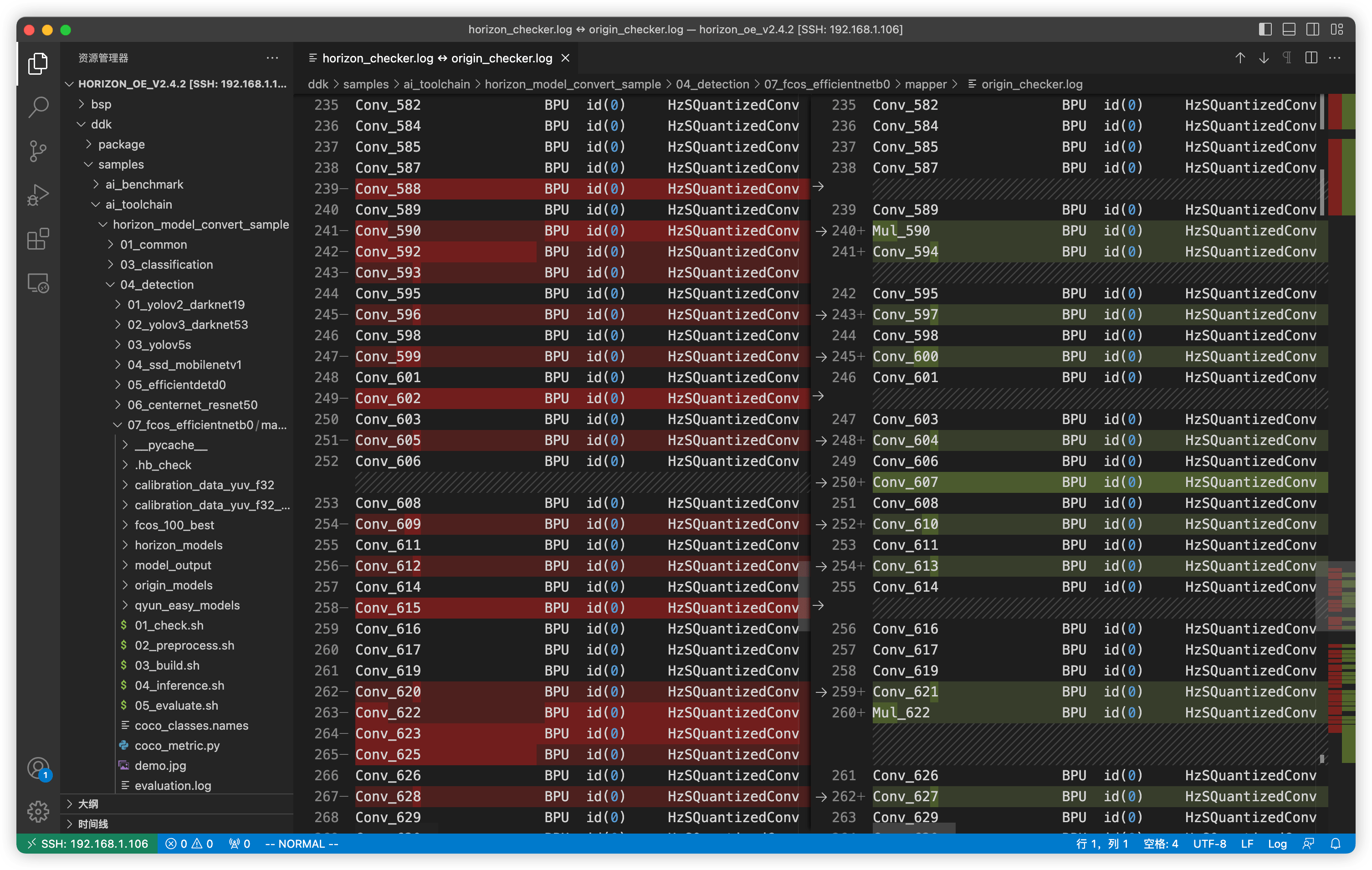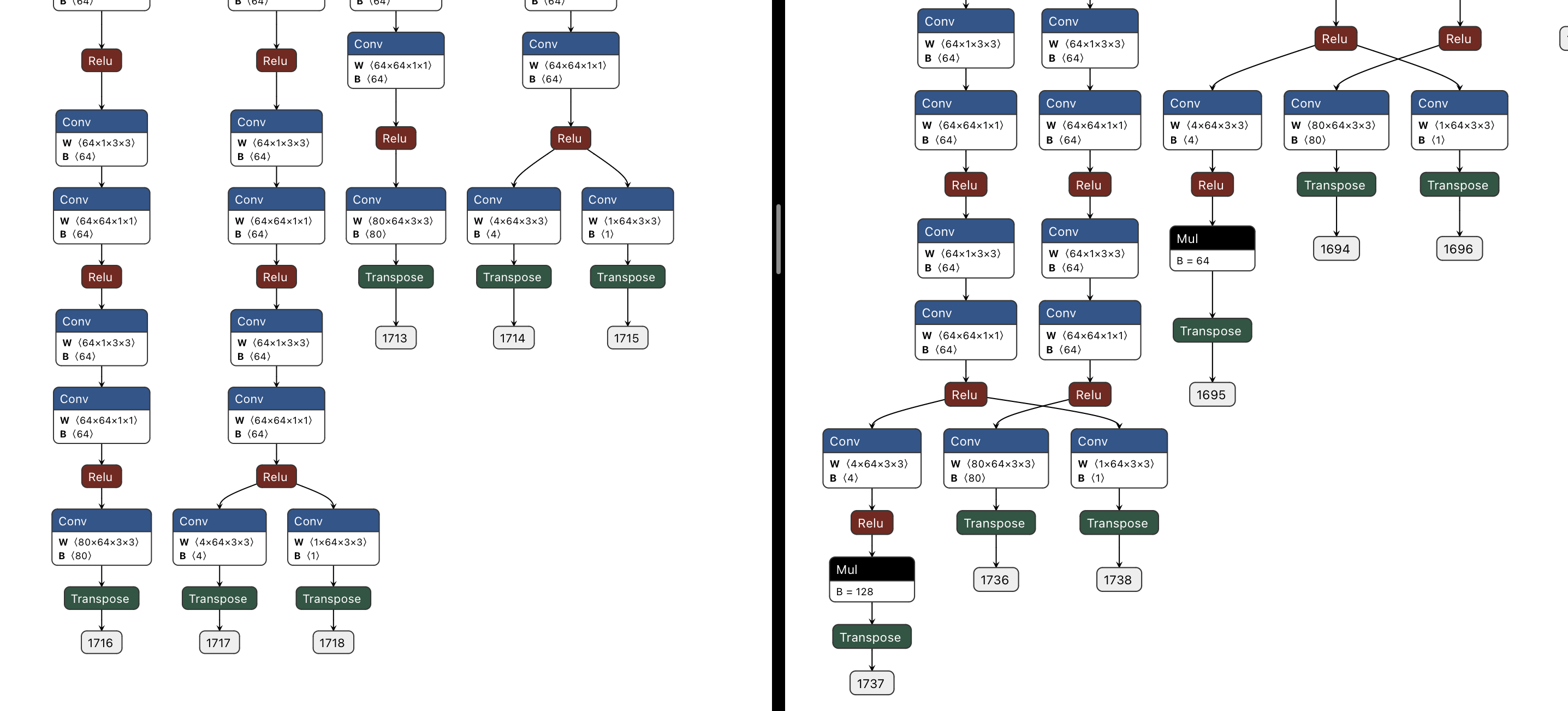``````# Decode 函数中获取数据的部分
# 各个 stride 找符合的模型
for i in range(len(strides)):
cls = outputs[i].buffer
bbox = outputs[i + 5].buffer * strides[i]  # modified
ce = outputs[i + 10].buffer
scores = _scores(cls, ce)
``````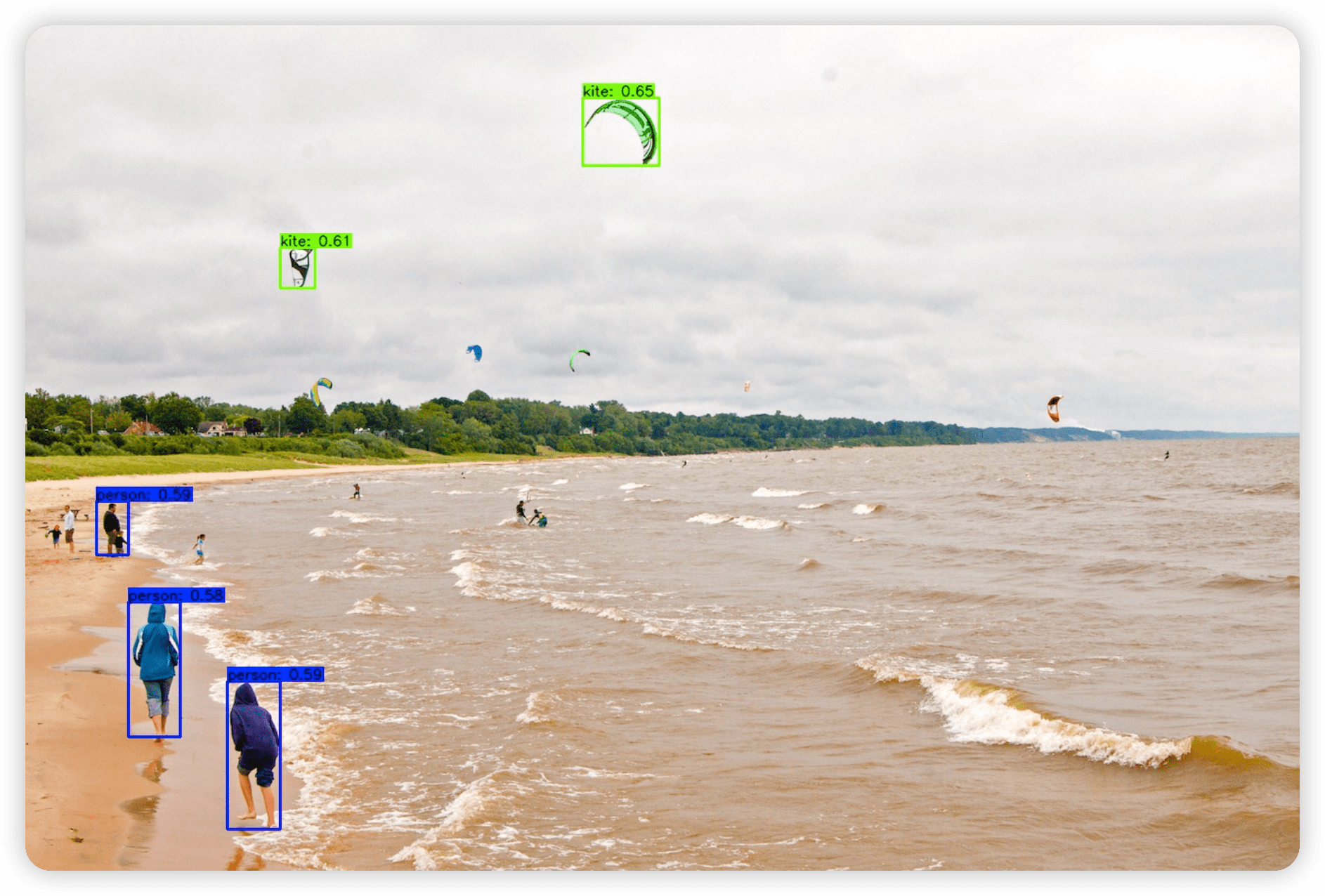## 训练自采集数据集#

### 数据准备#

``````[root@Z690-P selfcoco]# tree
.
├── annotations
│   ├── instances_train2017.json  # 训练集的 label
│   └── instances_val2017.json    # 验证集的 label
├── train2017   # 训练集照片
└── val2017     # 验证集照片
``````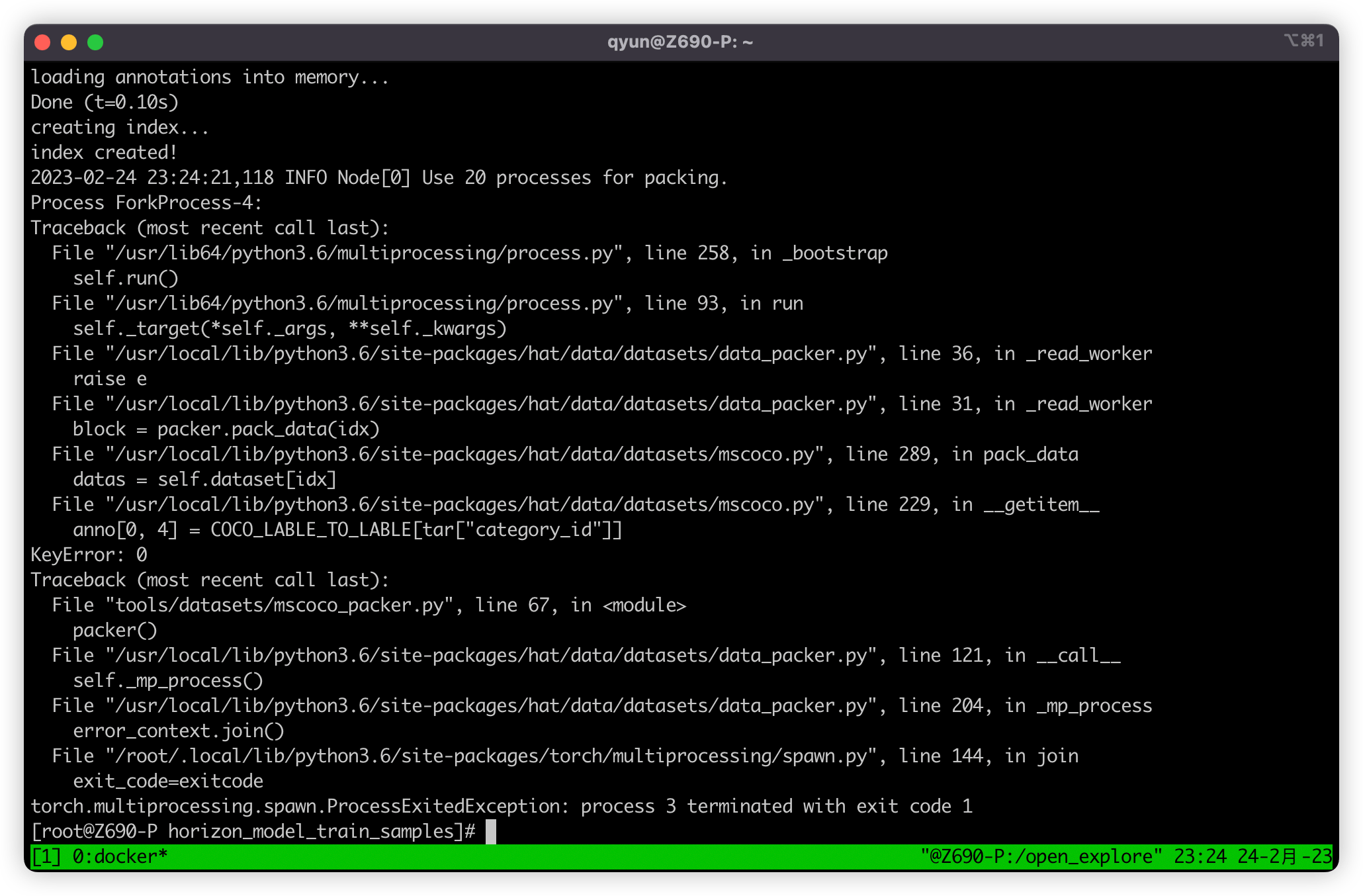Btw：🤣 这里的 COCO_LABLE_TO_LABLE 是不是拼错了，要拼 LABEL 的？

``````# 229 行
# anno[0, 4] = COCO_LABLE_TO_LABLE[tar["category_id"]]
anno[0, 4] = tar["category_id"]
# 327 行
# np.array([[COCO_LABLE_TO_LABLE[tar["category_id"]]]])
np.array([[tar["category_id"]]])
``````

``````# 转换训练集
python3 tools/datasets/mscoco_packer.py --src-data-dir /data/selfcoco --target-data-dir /data/selfcoco --split-name train --pack-type lmdb
# 转换验证集
python3 tools/datasets/mscoco_packer.py --src-data-dir /data/selfcoco --target-data-dir /data/selfcoco --split-name val --pack-type lmdb
``````

### 配置文件修改#

1. `num_classes`：这里就是一个全局变量类别数，例如我这里有五类 [0, 5)，那改为 5 即可。
2. `model`：model 参数中还有很多涉及到 80 的，经过我查看源码后，发现这些 80 的位置都应该改成 num_classes 参数才可以，例如 model 中的 loss_cls 参数，其中的 num_classes 参数值 80+1 就需要改为 `num_classes + 1` ，post_process 参数中的 num_classes 参数值 80 也应该改为 `num_classes`，这里我认为是官方的疏忽，应该是忘记改了。

``````# 进入 horizon_model_train_samples 目录
cd /open_explorer/ddk/samples/ai_toolchain/horizon_model_train_samples
# 开始训练
python3 tools/train.py --step float --config configs/detection/fcos/fcos_efficientnetb0_mscoco.py
``````

### 校准数据准备#

``````# 02_preprocess.sh
set -e -v

cd \$(dirname \$0) || exit

python3 ../../../data_preprocess.py \
--src_dir /data/selfcoco/train2017 \ # 重要
--dst_dir ./calibration_data_yuv_f32 \
--cal_img_num 50 \
--pic_ext .yuv \
--saved_data_type float32
``````

### 推理代码修改#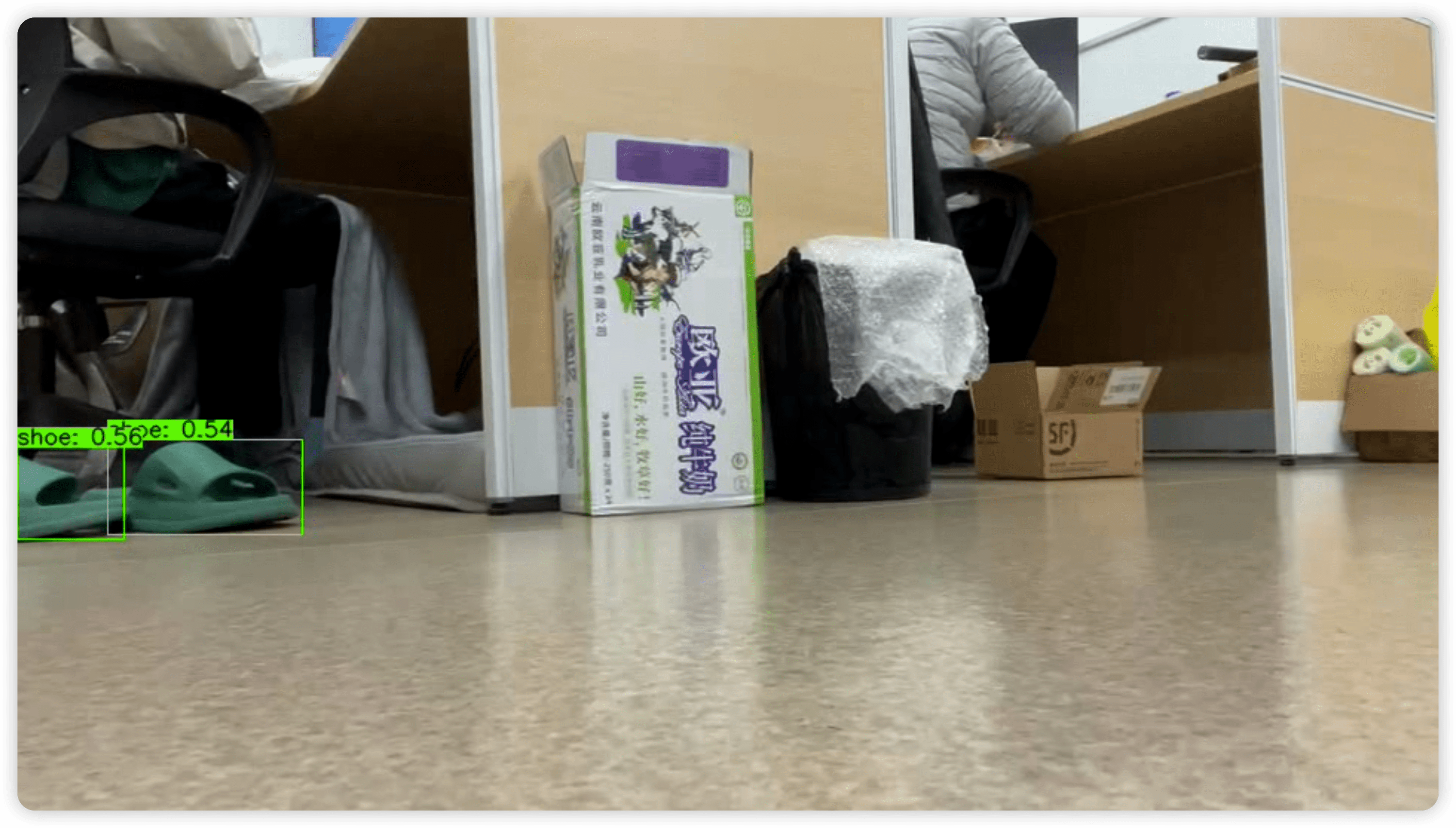## 后言#

1. 如何使用 CPU 进行训练而不是 GPU？
2. 如何基于已有的模型进行迁移训练？中断训练后可以再继续进行吗？
3. 可以 freeze 一部分参数进行 fine-tuning 吗？
4. 训练时有数据增强吗？如果不需要可以去掉吗？